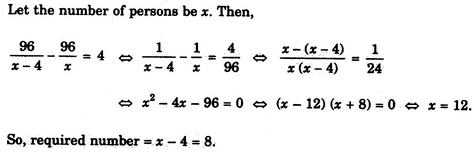# Verbal Reasoning - Arithmetic Reasoning

Exercise : Arithmetic Reasoning - Section 1
1.
The total of the ages of Amar, Akbar and Anthony is 80 years. What was the total of their ages three years ago ?
71 years
72 years
74 years
77 years
Explanation:

Required sum = (80 - 3 x 3) years = (80 - 9) years = 71 years.

2.
Two bus tickets from city A to B and three tickets from city A to C cost Rs. 77 but three tickets from city A to B and two tickets from city A to C cost Rs. 73. What are the fares for cities B and C from A ?
Rs. 4, Rs. 23
Rs. 13, Rs. 17
Rs. 15, Rs. 14
Rs. 17, Rs. 13
Explanation:

Let Rs. x be the fare of city B from city A and Rs. y be the fare of city C from city A.

Then, 2x + 3y = 77 ...(i) and

3x + 2y = 73 ...(ii)

Multiplying (i) by 3 and (ii) by 2 and subtracting, we get: 5y = 85 or y = 17.

Putting y = 17 in (i), we get: x = 13.

3.
An institute organised a fete and 1/5 of the girls and 1/8 of the boys participated in the same. What fraction of the total number of students took part in the fete ?
2/13
13/40
None of these
Explanation:
No answer description is available. Let's discuss.

4.
A number of friends decided to go on a picnic and planned to spend Rs. 96 on eatables. Four of them, however, did not turn up. As a consequence, the remaining ones had to contribute Rs. 4 each extra. The number of those who attended the picnic was
8
12
16
24
Explanation:5.
A, B, C, D and E play a game of cards. A says to B, "If you give me three cards, you will have as many as E has and if I give you three cards, you will have as many as D has." A and B together have 10 cards more than what D and E together have. If B has two cards more than what C has and the total number of cards be 133, how many cards does B have ?
22
23
25
35
Explanation:

Clearly, we have :

B-3 = E ...(i)

B + 3 = D ...(ii)

A+B = D + E+10 ...(iii)

B = C + 2 ...(iv)

A+B + C + D + E= 133 ...(v)

From (i) and (ii), we have : 2 B = D + E ...(vi)

From (iii) and (vi), we have : A = B + 10 ...(vii)

Using (iv), (vi) and (vii) in (v), we get:

(B + 10) + B + (B - 2) + 2B = 1335B = 125B = 25.